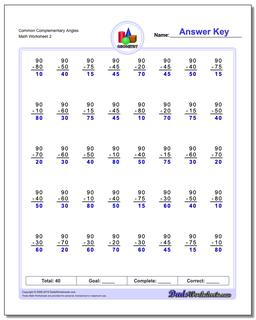# Math Worksheets: Basic Geometry: Basic Geometry: Common Complementary Angles (Second Worksheet)## Common Complementary Angles (Second Worksheet)

PropertyValue
DescriptionCommon Complementary Angles: Geometry worksheets with subtraction problems for finding complementary angles (Angles whose sum is 90 degrees). (Second Worksheet)
Resource TypeWorksheet